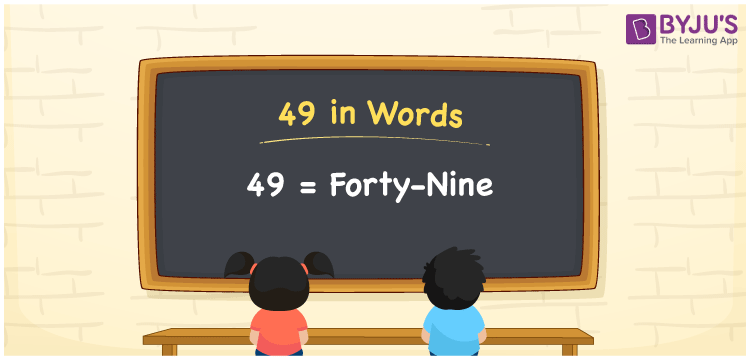# 49 in Words

49 in words is written as Forty-nine. The number 49 tells the count of objects or anything countable; hence it is a counting number. The numeral 49 represents a value that is used in Maths and in real-life in many different ways. For example, while writing 49 rupees in a cheque, we can write Forty-nine only or Rupees Forty-nine only. By this example, we can understand how important it is to learn the numbers in words in the primary classes. Let us see in this article how to write 49 in words.

 49 in words Forty-nine Forty-nine in Numbers 49

## 49 in English Words

We generally write numbers in words using the English alphabet. Thus, we can read 49 in English as “Forty-nine”.## How to Write 49 in Words?

To convert 49 in words, we need to check the place value of its digits. Since 49 is a two-digit number, thus, place value of 4 is tens and 9 is ones. The order of place values of digits in a number is in accordance with the Indian numbering system, such as:

• Ones
• Tens
• Hundreds
• Thousands
• Ten-thousands
• Hundred-thousands or Lakhs

This can be done as follows.

 Tens Ones 4 9

This can be written as:

4 × Ten + 9 × One

= 4 × 10 + 9 × 1

= 40 + 9

= 49

= Forty-five

Thus, 49 in words is written as Forty-nine.

49 is a natural number that precedes 50 and succeeds 48.

49 in words – Forty-nine

Is 49 an odd number? – Yes

Is 49 an even number? – No

Is 49 a perfect square number? – Yes

Is 49 a perfect cube number? – No

Is 49 a prime number? – No

Is 49 a composite number? – Yes

## Frequently Asked Questions on 49 in Words

Q1

### How do you write 49 in English?

We can write 49 in English words as “Forty-nine”.
Q2

### What is 249 in English?

249 is written as Two hundred and forty-nine in English.
Q3

### Is 49 an even number or an odd number?

49 is an odd number since it is not completely divisible by 2.